# DAY Function – Get Day Number – Excel, VBA, Google Sheets

This tutorial demonstrates how to use the Excel DAY Function in Excel to get the day of the month.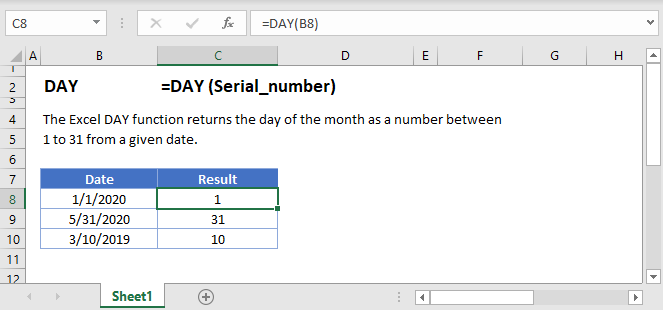## DAY Function Description:

The DAY Function Returns the day as a number (1-31) from a date.

To use the DAY Excel Worksheet Function, Select cell and Type: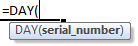(Notice how the formula input appear)

## DAY Function syntax and Inputs:

serial_number – The date in Excel serial number format or entered as a date with quotations (“s) surround the date. Example: You can not enter 11/12/2015 directly into the cell. Instead you need to enter “11/12/2015” or you would need to use the corresponding serial number: 42320. Alternatively, you can reference a cell with the date 11/12/2015 entered. Excel automatically converts dates stored in cells into serial format (unless the date is entered as text).

## DAY Examples

The DAY Function returns the day number of a date: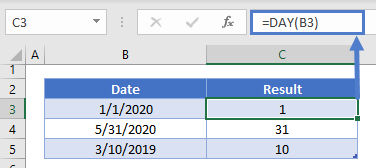### Day Number of Year

To calculate the day number of the year subtract the first day of the year from your desired date.  Do this by using the DATE and YEAR Functions: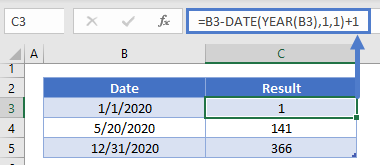### DAY and MONTH Functions

Using the DATE and DAY Functions you can build a new date using the same day number as the original date: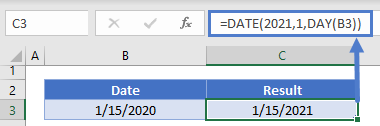The DAY Function works exactly the same in Google Sheets as in Excel: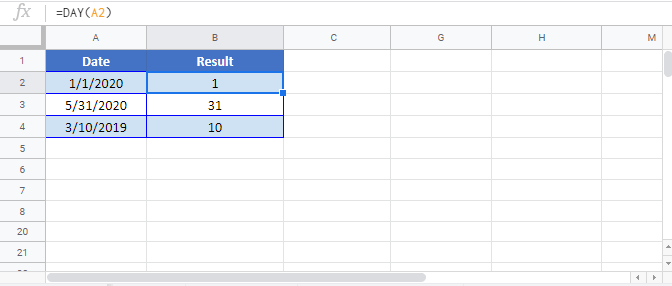## DAY Examples in VBA

You can also use the DAY function in VBA. Type:
`application.worksheetfunction.day(serial_number)`
For the function arguments (serial_number, etc.), you can either enter them directly into the function, or define variables to use instead.